Module 7 - Answers Lesson 1 Answer 1 7.1.1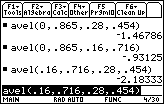The average velocity from t = 0 to t = 0.16 was –0.93125. The average velocity from t = 0.16 to t = 0.28 was –2.18333.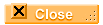Answer 2 7.1.2   The book fell faster during the subinterval from t = 0.16 to t = 0.28.Lesson 2 Answer 1 7.2.1 Average velocities: –2.1 m/s; –1.933 m/s; –1.75 m/s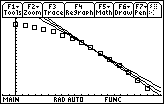Answer 2 7.2.2 The best approximation is –1.75 m/s, which is the average velocity for the interval (0.16, 0.716) to (0.18, 0.681). It best approximates the instantaneous velocity because the elapsed time interval is the smallest of the three.Answer 3 7.2.3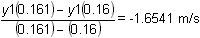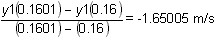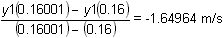Lesson 3 Answer 1 7.3.1 –1.64509 m/s; –1.64914 m/s; –1.64955 m/sAnswer 2 7.3.2 Yes. This average velocity is also –1.6496 m/s, which is equal to the instantaneous velocity.Self Test Answer 1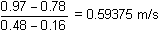The ball averaged approximately 0.6 m/s over the interval from t = 0.16 to t = 0.48, traveling in an upward direction. Answer 2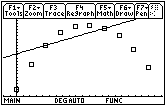Answer 3 2.1401 m/s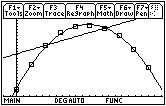Answer 4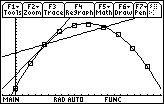Answer 5 2.1447 m/s Answer 6 2.1447 m/s; The ball was traveling approximately 2.1447 m/s at t = 0.16 in an upward direction.©Copyright 2007 All rights reserved. | Trademarks | Privacy Policy | Link Policy